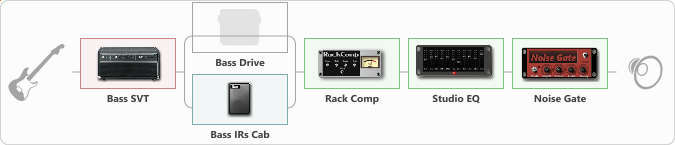# Bass Drive

Discussion in 'ToneLib-GFX presets' started by Lastik29ru, Jun 1, 2021.

1. Bass Dr

Preset name: Bass Dr

Effects chain:Effect: "Bass SVT" (Усилители симуляторы), active - "yes"
{
"Bass Ctrl" = UltraLo
"Midrange" = 800 Hz
"Ultra Hi" = On
"Bass" = 4
"Middle" = 3
"Treble" = 2
"Volume" = 70
"Level (dB)" = 1
}

Effect: "Splitter" (Динамика / Фильтр)
{
"A-Bypass" = Off
"A-Pan" = 0
"A-Level" = 55
"B-Bypass" = Off
"B-Pan" = 0
"B-Level" = 55

'A' branch:
{

Effect: "Bass Drive" (Перегрузка / Искажение), active - "no"
{
"Drive" = 76
"Blend" = 20
"Presence" = 21
"Bass" = 75
"Middle" = 25
"Treble" = 42
"Level" = 78
}
}
'B' branch:
{

Effect: "Bass IRs Cab" (Кабинеты), active - "yes"
{
"Model" = Ampeg SVT 810e (8x10")
"Mic Position" = Middle
"Mic Distance" = Middle
"Low Cut (Hz)" = 30
"Hi Cut (kHz)" = 7.0
"Mix" = 100
"Level (dB)" = 0
}
}
}

Effect: "Rack Comp" (Динамика / Фильтр), active - "yes"
{
"Threshold (dB)" = -24
"Ratio" = 1
"Attack" = Slow
"Release (ms)" = 1000
"Knee" = 50
"Level (dB)" = 4
}

Effect: "Studio EQ" (Динамика / Фильтр), active - "yes"
{
"31 Hz" = 0
"62 Hz" = -1
"125 Hz" = 7
"250 Hz" = 2
"500 Hz" = -1
"1 kHz" = 0
"2 kHz" = 2
"4 kHz" = 1
"8 kHz" = -2
"16 kHz" = -4
"Level (dB)" = 0
}

Effect: "Noise Gate" (Динамика / Фильтр), active - "yes"
{
"Mode" = Auto
"Depth" = 31
"Threshold" = 58
"Attack" = 88
"Hold" = 995
"Decay" = 665
}

Note: You will need to download and install the ToneLib-GFX software to use the preset.